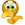# Nested IF Statement - Subtract Weekend Days

## Excel Help for Nested If Statement - Subtract Weekend Days in Analyzing Corporate Financial Data

### Nested If Statement - Subtract Weekend Days

Rate this:
(3/5 from 1 vote)I use IF statements to track invoices from approval date to mail date.  The following statement is used to track the # of days it takes to finalize and invoice once the invoice is approved: =IF(ISBLANK(Q45),"",Q45-K45)  Q45 represents the date the invoice was processed and K45 represents the approval date.  Does anyone know a way to adjust the formula so weekends are not included in the total?? i.e if a weekend fell in between the approval date and the process date, the formula would subtract 2 from the total...?? Please help!!! Posted by RossPQ on 02 Feb 2011
 Replies - Displaying 1 to 6 of 6 Order Replies By: Most recent | Chronological | Highest RatedRate this: (3/5 from 1 vote) The NETWORKDAYS function worked beautifully.  Thank you all Posted by RossPQ on 03 Feb 2011Rate this: (3/5 from 1 vote) Yes - the NETWORKDAYS function is a much simpler solution.  If you are running prior to Excel 2007 (e.g. Excel 2003), you will need to install the Analysis ToolPak to get this function available. Excel Business Forums Administrator Posted by Excel Helper on 03 Feb 2011Rate this: (3/5 from 1 vote) =networkdays(startdate,enddate) Posted by Roshan10043 on 03 Feb 2011Rate this: (3/5 from 1 vote) This is the formula I tried:=IF(ISBLANK(Q45),"",IF(WEEKDAY(Q45)<=WEEKDAY(K45),Q45-K45-2,OR(IF(WEEKDAY(Q45+7)<=WEEKDAY(K45),Q45-K45-4,Q45-K45))))It only subtracts 2 in any scenario.  Any suggestions??Thanks Posted by RossPQ on 03 Feb 2011Rate this: (3/5 from 1 vote) Thank you for the response - it was helpful.What if the dates are greater than one week apart?  i.e an invoice was approved on Jan 6 and processed on Jan 18.  In this scenario, I would like to subtract 4 from the total.  Is there a condition that could be added to the formula to account for this?  Possibly:IF(WEEKDAY(Q45+7)<=WEEKDAY(K45),Q45-K45-4Again, I would like this included in the original formula so that the formula is constant throughout the spreadsheet.Thanks again! Posted by RossPQ on 03 Feb 2011Rate this: (4/5 from 2 votes) You can use the WEEKDAY function on the dates which will return 7 for Sunday and 1 for Saturday.  To test if the weekend falls between the 2 dates that are within one week apart, the formula would be:=IF(ISBLANK(Q45),"",IF(WEEKDAY(Q45)<=WEEKDAY(K45),Q45-K45-2,Q45-K45)) Excel Business Forums Administrator Posted by Excel Helper on 02 Feb 2011
 Displaying page 1 of 1

 Find relevant Excel templates and add-ins for Nested IF Statement - Subtract Weekend Days in the Excel Business Solutions Directory